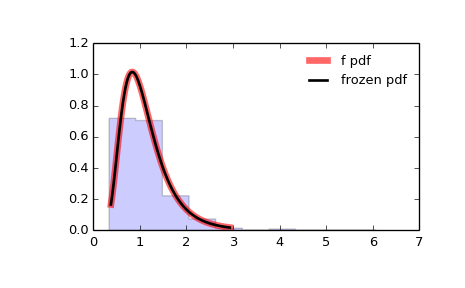# scipy.stats.f¶

scipy.stats.f = <scipy.stats._continuous_distns.f_gen object at 0x450470ac>[source]

An F continuous random variable.

As an instance of the rv_continuous class, f object inherits from it a collection of generic methods (see below for the full list), and completes them with details specific for this particular distribution.

Notes

The probability density function for f is:

                     df2**(df2/2) * df1**(df1/2) * x**(df1/2-1)
F.pdf(x, df1, df2) = --------------------------------------------
(df2+df1*x)**((df1+df2)/2) * B(df1/2, df2/2)


for x > 0.

f takes dfn and dfd as shape parameters.

The probability density above is defined in the “standardized” form. To shift and/or scale the distribution use the loc and scale parameters. Specifically, f.pdf(x, dfn, dfd, loc, scale) is identically equivalent to f.pdf(y, dfn, dfd) / scale with y = (x - loc) / scale.

Examples

>>> from scipy.stats import f
>>> import matplotlib.pyplot as plt
>>> fig, ax = plt.subplots(1, 1)


Calculate a few first moments:

>>> dfn, dfd = 29, 18
>>> mean, var, skew, kurt = f.stats(dfn, dfd, moments='mvsk')


Display the probability density function (pdf):

>>> x = np.linspace(f.ppf(0.01, dfn, dfd),
...                 f.ppf(0.99, dfn, dfd), 100)
>>> ax.plot(x, f.pdf(x, dfn, dfd),
...        'r-', lw=5, alpha=0.6, label='f pdf')


Alternatively, the distribution object can be called (as a function) to fix the shape, location and scale parameters. This returns a “frozen” RV object holding the given parameters fixed.

Freeze the distribution and display the frozen pdf:

>>> rv = f(dfn, dfd)
>>> ax.plot(x, rv.pdf(x), 'k-', lw=2, label='frozen pdf')


Check accuracy of cdf and ppf:

>>> vals = f.ppf([0.001, 0.5, 0.999], dfn, dfd)
>>> np.allclose([0.001, 0.5, 0.999], f.cdf(vals, dfn, dfd))
True


Generate random numbers:

>>> r = f.rvs(dfn, dfd, size=1000)


And compare the histogram:

>>> ax.hist(r, normed=True, histtype='stepfilled', alpha=0.2)
>>> ax.legend(loc='best', frameon=False)
>>> plt.show()Methods

 rvs(dfn, dfd, loc=0, scale=1, size=1, random_state=None) Random variates. pdf(x, dfn, dfd, loc=0, scale=1) Probability density function. logpdf(x, dfn, dfd, loc=0, scale=1) Log of the probability density function. cdf(x, dfn, dfd, loc=0, scale=1) Cumulative density function. logcdf(x, dfn, dfd, loc=0, scale=1) Log of the cumulative density function. sf(x, dfn, dfd, loc=0, scale=1) Survival function (1 - cdf — sometimes more accurate). logsf(x, dfn, dfd, loc=0, scale=1) Log of the survival function. ppf(q, dfn, dfd, loc=0, scale=1) Percent point function (inverse of cdf — percentiles). isf(q, dfn, dfd, loc=0, scale=1) Inverse survival function (inverse of sf). moment(n, dfn, dfd, loc=0, scale=1) Non-central moment of order n stats(dfn, dfd, loc=0, scale=1, moments='mv') Mean(‘m’), variance(‘v’), skew(‘s’), and/or kurtosis(‘k’). entropy(dfn, dfd, loc=0, scale=1) (Differential) entropy of the RV. fit(data, dfn, dfd, loc=0, scale=1) Parameter estimates for generic data. expect(func, dfn, dfd, loc=0, scale=1, lb=None, ub=None, conditional=False, **kwds) Expected value of a function (of one argument) with respect to the distribution. median(dfn, dfd, loc=0, scale=1) Median of the distribution. mean(dfn, dfd, loc=0, scale=1) Mean of the distribution. var(dfn, dfd, loc=0, scale=1) Variance of the distribution. std(dfn, dfd, loc=0, scale=1) Standard deviation of the distribution. interval(alpha, dfn, dfd, loc=0, scale=1) Endpoints of the range that contains alpha percent of the distribution

#### Previous topic

scipy.stats.exponpow

#### Next topic

scipy.stats.fatiguelife